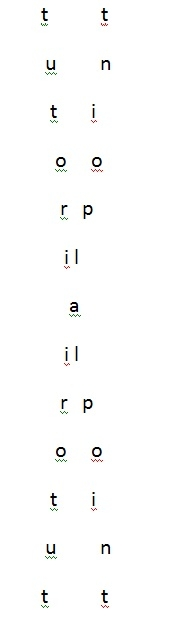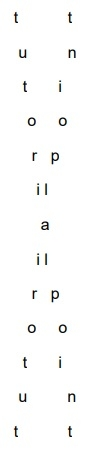# Print string of odd length in ‘X’ format in C Program.

CServer Side ProgrammingProgramming

#### C in Depth: The Complete C Programming Guide for Beginners

45 Lectures 4.5 hours

#### Practical C++: Learn C++ Basics Step by Step

Most Popular

50 Lectures 4.5 hours

#### Master C and Embedded C Programming- Learn as you go

66 Lectures 5.5 hours

Given with a string program must print the string in ‘X’ format. For reference, see the image given below.Here, one variable can be used to print from left right(“i”) and other variable can used to print from right to left(“j”) and we can take other variable k which is used for space calculation.

Below is the C++ implementation of the algorithm given.

## Algorithm

START
Step 1 ->Declare Function void print(string str, int len)
Loop For int i = 0 and i < len and i++
Set int j = len-1- i
Loop For int k = 0 and k < len and k++
IF k == i || k == j
Print str[k]
End
Else
Print " "
End
End
Step 2 -> In main()
Declare string str = "tutorialpoint"
Set int len = str.size()
Call print(str, len)
STOP

## Example

#include<iostream>
using namespace std;
void print(string str, int len){
for (int i = 0; i < len; i++){
int j = len-1- i;
for (int k = 0; k < len; k++){
if (k == i || k == j)
cout << str[k];
else
cout << " ";
}
cout << endl;
}
}
int main (){
string str = "tutorialpoint";
int len = str.size();
print(str, len);
return 0;
}

## Output

if we run above program then it will generate following output